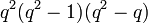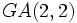GA(2,2) is isomorphic to S4

(diff) ← Older revision | Latest revision (diff) | Newer revision → (diff)
This article gives a proof/explanation of the equivalence of multiple definitions for the term symmetric group:S4
View a complete list of pages giving proofs of equivalence of definitions

Statement

The general affine group of degree two over field:F2 (the field of two elements) is isomorphic to symmetric group:S4.

Facts used

1. Order formulas for linear groups of degree two

Proof

Step no. Assertion/construction Facts used Previous steps used Explanation
1 For any field$k$ the group$GA(2,k)$ has a faithful group action on$k^2$ and hence has an injective homomorphism to the symmetric group on$k^2$. By definition of$GA(n,k)$, it has a faithful group action on$k^n$.
2 For a field of size$q$,$GA(2,q) = GA(2,\mathbb{F}_q)$ has size$q^2(q^2 - 1)(q^2 - q)$. Fact (1) [SHOW MORE]
3 For$k$ the field of size two, the symmetric group on$k^2$ is the symmetric group of degree four and its order is 24, and$GA(2,k)$ has order$2^2(2^2 - 1)(2^2 - 2) = 24$. Step (2) [SHOW MORE]
4 For$k$ the field of size two, the injective homomorphism of Step (2) gives an isomorphism from$GA(2,2)$ to$S_4$. Steps (1), (3) [SHOW MORE]